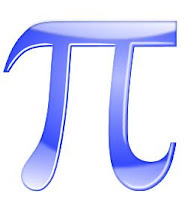## Tuesday, 5 June 2012

### NCERT Chapter SolutionsIs Pi rational or irrational? (src:openclipart.org)
Q1: State whether the following statements are true or false. Justify your answers.

(i) Every irrational number is a real number. (TRUE)
Explanation: Real numbers are the collection of rational and irrational numbers.

(ii) Every point on the number line is of the form  √m, where m is a natural number. (FALSE)
Explanation:  Since -ve numbers cannot be expressed as square roots.

(iii) Every real number is an irrational number. (FALSE)
Explanation: Real numbers contains both rational and irrational numbers.

Q2. Are the square roots of all positive integers irrational? If not, give an example of the square root of a number that is a rational number.

Answer: No, the  square roots of all positive integers can be rational or irrational. e.g. √9 = 3 which is a rational number.

Q3: Show how √5 can be represented on the number line.

Answer:  We can locate n for any positive integer n, after n − 1 has been located.
We can write √5 in Pythagoras form:

= √(4 + 1) = √(22 + 1)
Construction:
1. Take a line segment AB = 2 units (consider 1 unit = 2 cm) on x-axis.
2. Draw a perpendicular on B and construct a line BC = 1 unit length.
3. Join AC which will be √5 (Pythagoras theorem). Take A as center, and AC as radius draw an which cuts the x-axis at point E.
4. The line segment AC represents √5 units.

Q4. Construct the ‘square root spiral’ (Class room activity.)

Following video shows the square root spiral.

Q5: Who discovered √2  or disclosed its secret?

It is assumed Pythagoreans, followers of the famous Greek mathematician Pythagoras, were the first to discover the numbers which cannot be written in the form of a fraction. These numbers are called irrational numbers.

Q6: Who showed that showed that "Corresponding to every real number, there is a point on the real number line, and corresponding to every point on the number line, there exists a unique real number"?

Answer: Two German mathematicians, Deddkind and Cantor.

Q6: Is π (pi) rational or irrational?

Answer: π is an irrational number. 22/7 is just an approximation.

Q7: How many rational numbers exist between any two rational numbers?

Q8: Are irrational numbers finite?

Answer: No. There are infinite set of irrational numbers.

Q9: The product of any two irrational numbers is :
(A) always an irrational number.
(B) always a rational number.
(C) always an integer.
(D) sometimes rational, sometimes irrational number.

Answer: (D) sometimes rational, sometimes irrational number
e.g. √2 and √3 are two irrational numbers, √2×√3 = √6 an irrational number.

√2 and √8 are two irrational numbers, √2×√8 = √(16) = 4 a rational number.1.great,,,
i want to share about irrational number

http://www.math-worksheets.co.uk/048-tmd-what-are-irrational-numbers/

2.i want the solution for this question of rationalisation
sq root of (3+2*sq root of2)

1.3.4.Ok thank you

5.6.